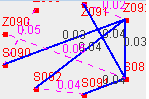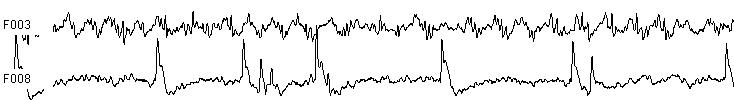Brain Graph Youcan
 Determing a Hamiltonian cycle or not in bipartite cubic graph is polynomial Guilin University of Electronic Technology No.1 Jinji Road, Guilin, 541004, China The main theorem is shown in follow. THEOREM 1 Given a bicubic graph G, Determining a Hamiltonian cycle exists or not is no more than O(n^3.5) time. Determining a Hamiltonian cycle in 2-connected bipartite cubic graph is a NP-completed problem . Although I had proved the Hamiltonian cycle problem on degree bound two is Polynomial . Unfortunately, it is hard to reduce the cubic graph to the digraph graph with degree bound two by simple substitute the edge into two symmetric arcs, since the in out degree both are 3. Our approach is to reduce the bipartite cubic graph into a 2-factor and a perfect matching, which comes from the petersen theorem. THEOREM 2  bridgeless cubic bipartite graph has a 1-factor and hence have a 2-factor. Since bipartite cubic graph G include a 2-factor and a perfect matching. Then it is easy to obtain follows lemma. Lemma 1 Given a bridgeless bipartite cubic graph G, assume the M is a perfect matching of G. then G-M is a 2-factor graph. According to the principle of reverse function of Z-mapping in . It is easy to deduce that. Lemma 2 Given a bridgeless bipartite cubic graph G and a perfect matching M , then the reverse function of z-mapping is a digraph \$D\$ with degree bound two. Thus, it is easy to deduce that Lemma 3 if \$D\$ is Hamiltonian, then \$G\$ is Hamiltonian. This is a sufficient condition for bipartite cubic graph. The sufficient and necessary conditions will be described soon. I implement the idea with C++ under Linux and Windows. Now we given the binary distribution file as following. Linux : hcpbicubic (which had been test under slackware 10.0) Windows: Hcpbicubic.exe (which had been test under Windows2000, Windows XP ) How to use the tool. Firstly, it needs to write the adjacency matrix of a given graph. Then you should create a text file include the adjacency matrix as follows. Input data format: First line: From second line: adjacency matrix of Graph. Test bench Testing data We selected some famous graph, one of test data is shown very hard for many availed tools (as far as we known). NonHamiltonian graph: Horton96.txt , 50cubic.txt  Hamiltonian graph: 96h.txt , 50h.txt Reference:  T. Akiyama, T. Nishizeki, N. Saito, NP-Completeness of the Hamiltonian Cycle Problem for Bipartite Graphs. Journal of Information Processing, Vol. 3 No. 2, (1980), 73--76  Guohun Zhu, The Complexity of HCP in Digraphs with Degree Bound Two . arXiv:0704.0309v3 Guohun Zhu. The Complexity of Determining Existence a Hamiltonian Cycle is \$O(n^3)\$. arXiv:0706.2725  J. Petersen, Die Theorie der regul?ren Graphen, Acta Math. 15 (1891), 193¨C220.  Bondy, J. A. and Murty , U. S. R. Graph Theory with Applications. New York : North Holland , pp. 61 and 240, 1976.  Branko GrÄunbaum. 3-connected configurations (n3) with no Hamiltonian circuit. Bull. Inst. Combin. Appl., 46:15-26, 2006.